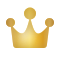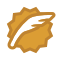# 「信息安全-密码与隐藏技术」RSA加密算法的实现（CPP 实现）

## RSA加密算法的实现

### 关键代码：求逆元

``// 拓展gcdvoid exgcd(ll a,ll b,ll& d,ll& x,ll& y){if(!b) { d = a; x = 1; y = 0; }else{ exgcd(b, a%b, d, y, x); y -= x*(a/b); }}// 求逆元ll inv(ll a, ll p){ll d,x,y;exgcd(a,p,d,x,y);return d == 1 ? (x+p)%p : -1;}``

## 关键代码：平方-乘算法

``#include<bits/stdc++.h>#define mst(a,b) memset(a,b,sizeof(a);#define INF 0x3f3f3f3fusing namespace std;typedef long long ll;// ¿ìËÙÃÝll poww(ll a,ll b,ll mod){ll ans=1,base=a;while(b){if(b&1)ans = ans*base%mod;base = base*base%mod;b>>=1;}return ans;}int main(){cout<<poww(34,60,51)<<endl; //34cout<<poww(345,89,101)<<endl; //34}``

### 实现源码

``#include<bits/stdc++.h>#define mst(a,b) memset(a,b,sizeof(a);#define INF 0x3f3f3f3fusing namespace std;const int maxn=1e3+5;int n,m,t;typedef long long ll;// 拓展gcdvoid exgcd(ll a,ll b,ll& d,ll& x,ll& y){if(!b) { d = a; x = 1; y = 0; }else{ exgcd(b, a%b, d, y, x); y -= x*(a/b); }}// 求逆元ll inv(ll a, ll p){ll d,x,y;exgcd(a,p,d,x,y);return d == 1 ? (x+p)%p : -1;}// 快速幂ll poww(ll a,ll b,ll mod){ll ans=1,base=a;while(b){if(b&1)ans = ans*base%mod;base = base*base%mod;b>>=1;}return ans;}// 加密过程ll encryption(ll pk,ll r,ll num){return poww(num,pk,r)%r;}// 解密过程ll decrypt(ll sk,ll r,ll text){return poww(text,sk,r)%r;}int main(){ll prime1,prime2,pk;cout<<"请输入测试数据组数："<<endl;cin>>t;while(t--){cout<<"请输入两个大的素数和公钥"<<endl;cin>>prime1>>prime2>>pk;// 公开模数ll r = prime1 * prime2;// 欧拉函数ll n = (prime1-1)*(prime2-1);cout<<"公开模数r为："<<r<<endl;ll sk = inv(pk,n);cout<<"私钥sk为："<<sk<<endl;cout<<"请输入要加密的数字串（输入0结束）："<<endl;ll num;cin>>num;while(num){// 密文int cipherText = encryption(pk,r,num);cout<<"加密后的密文为："<<cipherText<<endl;// 明文int plainText = decrypt(sk,r,cipherText);cout<<"解密后的明文为："<<plainText<<endl;cin>>num;}}return 0;}``
``学如逆水行舟，不进则退``一百个ChocolateCSDN认证博客专家CSDN博客专家博客之星前端开发攻城狮学如逆水行舟，不进则退